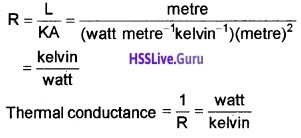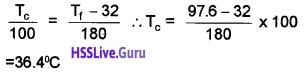# Plus One Physics Chapter Wise Questions and Answers Chapter 11 Thermal Properties of Matter

Students can Download Chapter 11 Thermal Properties of Matter Questions and Answers, Plus One Physics Chapter Wise Questions and Answers helps you to revise the complete Kerala State Syllabus and score more marks in your examinations.

## Kerala Plus One Physics Chapter Wise Questions and Answers Chapter 11 Thermal Properties of Matter

### Plus One Physics Thermal Properties of Matter One Mark Questions and Answers

Question 1.
In which of the following processes, convection does nottake place primarily?
(a) Sea and land breeze
(b) Boiling of water
(c) Warming of glass of bulb due to filament
(d) Heating air around a furnace
(c) Warming of glass of bulb due to filament
In convection process, the heat is transferred by the bodily motion of the heated particles. It is not so in case of warming of glass bulb due to filament heating. In fact, warming of glass bulb is due to radiation.

Question 2.
$$\frac{\text { Watt }}{\text { Kelvin }}$$ a unit of
(a) Stefan’s constant
(b) Wien’s constant
(c) Cooling’s constant
(d) Thermal conductance
(d) Thermal resistanceQuestion 3.
For measuring temperatures in the range of 2000°C, we should employ
(a) gas thermometer
(b) platinum-rhodium thermometer
(c) barometer
(d) pyrometer
(d) pyrometer

Question 4.
There is a hole in metal disc. What happens to the size of metal disc if the metal disc is heated?
The size of hole increases.

Question 5.
Which has more specific heat capacity, water, and sand?
Water.

Question 6.
Two solid spheres of the same material have the same radius but one is hollow while the other is solid. Both spheres are heated to same temperature. Then
(a) the solid sphere expands more
(b) the hollow sphere expands more
(c) expansion is same for both
(d) nothing can be solid about their relative expansion if their masses are not given
(c) expansion is same for bothQuestion 7.
The sprinkling of water reduces slightly the temperature of a closed room because
(a) temperature of water is less than that of the room.
(b) specific heat of water is high
(c) water has large latent heat of vaporisation
(d) water is a bad conductor of heat
(c) water has large latent heat of vaporisation
When water is sprinkled over a large area, evaporation takes place. As the latent heat of vaporisation is large cooling takes place.

Question 8.
Why specific heat of gas at constant pressure (Cp) is greater than specific heat at constant volume?
More heat is required to raise the temperature of gas at constant pressure than at constant volume.

Question 9.
A body is heated. But there is no change in its temperature. Is it possible?
Yes. During change of state, there will be no increase in temperature even when heat is supplied.

Question 10.
When boiling water is put in glass tumbler, the tumbler cracks. Why?
Glass is poor conductor of heat. So inner and outer surfaces of tumbler suffer uneven expension. Hence it breaks.

Question 11.
A small space is left between two rails on railway track. Why?
If no space is left, the rails would bend due to thermal expansion in summer. So small space is left between two rails to allow thermal expansion.Question 12.
The pendulum of clock is made of invar, Why?
Invar has low value of coefficient of linear expansion. So length of pendulum remains almost same in all seasons. (The change in length affects time period of pendulum).

Question 13.
Tea gets cooled, when sugar is added to it. Why?
When sugar is added, heat content of tea gets shared with sugar & hence’ temperature decreases.

Question 14.
Ice covered in gunny bag does not melt for a long time. Why?
A gunny bag is poor conduct of heat & hence it does not allow external heat to enter.

Question 15.
Why two layers of cloth of equal thickness provide warmer covering than a single layer of cloth of double the thickness?
Because air between two layers of clothes is a bad ‘ conductor of heat.Question 16.
On winter nights, we feel warmer when clouds cover the sky than the sky is clear. Why?
The clouds are bad conductor of heat. So heat of earth’s atmosphere is not conducted out.

### Plus One Physics Thermal Properties of Matter Two Mark Questions and Answers

Question 1.
1. Two bodies at different temperatures T1K and T2K are brought in contact with each other

• Is the resultant temperature be necessarily (T1 + T2)/2? If not, Why?
• Should the resultant temperature be between T1 and T1 only? If not, Why?

1. Two bodies at different temperatures:

• The resultant temperature may not be necessarily $$\left(\frac{T_{1}+T_{2}}{2}\right)$$. Because specific heat capacity is different for all substances.
• If heat is not lost to the surroundings, resultant temperature must lie in between T1 and T2.Question 2.
A Patient is admitted to hospital. The temperature of the patient is measured by nurse and is found to be 97.6°F

1. What is meant by temperature?
2. Convert the temperature (97.6°F) is to centigrade

1. Temperature is the degree of hotness.

2.Question 3.
Why iron rims are heated red hot before being put on the cart wheels?
The radius of iron rim is smaller than radius of cart wheel. When iron rim is heated, its radius increases due to thermal expansion. After rim has planted on the wheel, iron rim is allowed to cool. Then it fits tightly on the wheel due to thermal contraction.Question 4.
How woolen clothes helps us in winter against cold?
Wool is a heat insulator. Moreover, it contains a layer of dry air in it. This air, which is bad conductor of heat does not allow the body heat to escape & it also does not allow external cold to come in. The rough surface of woollen clothes absorbs more and reflects less heat falling on it.

### Plus One Physics Thermal Properties of Matter Three Mark Questions and Answers

Question 1.
A Solid material is supplied with heat at constant rate. The temperature of the material is changing with the heat input as shown in figure.

1. What do the horizontal region AB and CD represent?
2. What does the slope of DE represent?
3. The slope of OA is greater than the slope of BC. What does this indicate?1. Latent heat of fusion, Latent heat of vaporization
2. It indicates that, the material is in vapour state
3. It indicates that latent heat of vaporization of the material is greater than the latent heat of fusion.

### Plus One Physics Thermal Properties of Matter Four Mark Questions and Answers

Question 1.
A copper block of mass 2.5kg is heated in a furnace to a temperature of 500°C and then placed on a large ice block. What is the maximum amount of ice that can melt? (Specific heat of copper = 0.39Jg-1 K-1; latent heat of fusion of water = 335 Jg-1).
Mass, m = 2.5kg
= 2.5 × 103 g;
Change in temperature, ∆T = 500°C
Specific heat, c = 0.39 Jg-1K-1;
Latent heat of fusion, L = 335Jg-1
If m’ be the mass of ice melted, then m’L = mc ∆T
or m’ × 335 = 2.5 × 103 × 0.39 × 500 2.5 × 103 × 0.39 × 500
or m’ = $$\frac{2.5 \times 10^{3} \times 0.39 \times 500}{335} \mathrm{g}$$
= 1.5 kg.Question 2.
Heat from the sun reaches the earth.

1. Write the method of transmission of heat.
2. Thermal conductivity of Aluminium is 205 Jm-1s-1deg-1. What do you mean by this?
3. Birds swell feathers in winter. Why?

2. The coefficient of thermal conductivity of a substance is defind as the quauitity of heat conducted normally persecond through unit area of the substance per unit temperature gradient when the substance attains steady state.

3. By doing so the birds enclose air between the feathers. Air being a poor conductor, prevents the loss of heat from the body of the bird to the cold surroundings.

Question 3.
Two accidents are happened. The first one with water at 100°C and the second one with steam at 100°C.

1. Which is dangerous burn due to water at 100°C, and bum due to steam at 100°C? Why?
2. Latent heat of vapourisation of water to 536 cal/g. Explain the idea of latent heat of vopourisation.
3. Find the heat required to convert 1g of ice at 0°C to steam at 100°C is

1. Burn due to steam is more dangerous, because heat content in steam is very high compared to 100°C water.

2. Latent heat of vapourisation is the amount of heat required to change the state of 1 kg water in to vapour.

3. Q = ML + MC∆Q + ML1= 716 cal.Question 4.
When a fluid is heated, the particle rises up.

1. Name the phenomonon behind it.
2. Explain the formation of land breeze and sea breeze.

1. Convection

2. During the day, land heats up more quickly than, water in lake (due to high specific heat capacity of water). The air on the surface of earth gets heated, expands, becomes less dense and rises up. The colder air (wind) replaces the space created by hot air.

It creates a sea breeze. At night the land loses its heat very quickly than water. So water remains more warmer at night.

### Plus One Physics Thermal Properties of Matter NCERT Questions and Answers

Question 1.
A 10kW drilling machine is used to drill a bore in a small aluminium block of mass 8.0kg. How much is the rise in temperature of the block in 2.5 minutes, assuming 50% of power is used up in heating the machine itself or lost to the surroundings? Specific heat of aluminium = 0.19Jg-1 K-1.
Power, P = 10 kW
= 10 × 103W
= 104W
Mass, m = 8 kg; Time, t = 2.5min = 150s
Specific heat, c = 0.91Jg-1K-1
= 0.91 × 103Jkg-1K-1
Energy, Q = pt = 104 × 150J = 1.5 × 106J
It is given that 50% of energy is lost to the. surroundings. So, energy absorbed by the block is given by
Q = $$\frac{1}{2}$$ × 1.5 × 106J = 0.75 × 106J
But Q = mc∆T
∴ ∆TQuestion 2.
A brass boiler has a base area of 0.15m2 and thickness 1.0cm. It boils water at the rate of 6.0 kg/min when placed on a gas stove. Estimate the temperature of the part of the flame in contact with the boiler. Thermal conductivity of brass = 109 J s-1 m-1K-1;
Heat of vaporisation of water = 2256 × 103 J kg-1.
A = 0.15m2, d = 10-2m,
$$\frac{m}{t}=\frac{6}{60}$$ kgs-1 = 0.1kgs-1,
K = 109J s-1 m-1 K-1, L = 2256 × 103 Jkg-1,
θ1 = ?,
θ2 = 100°CQuestion 3.
Explain why?
(a) A body with large reflectivity is a poor emitter.
(b) A brass tumbler feels much colder than a wooden tray on a chilly day.
(c) An optical pyrometer (for measuring high temperature) calibrated for an ideal black body radiation gives too low a value for the temperature of a red hot iron piece in the open, but gives a correct value for the temperature when the same piece is in the furnace.
(d) The earth without its. atmosphere would be inhospitably cold?
(e) Heating systems based on circulation of steam are more efficient in warming a building than heating system based on circulation of hot water.